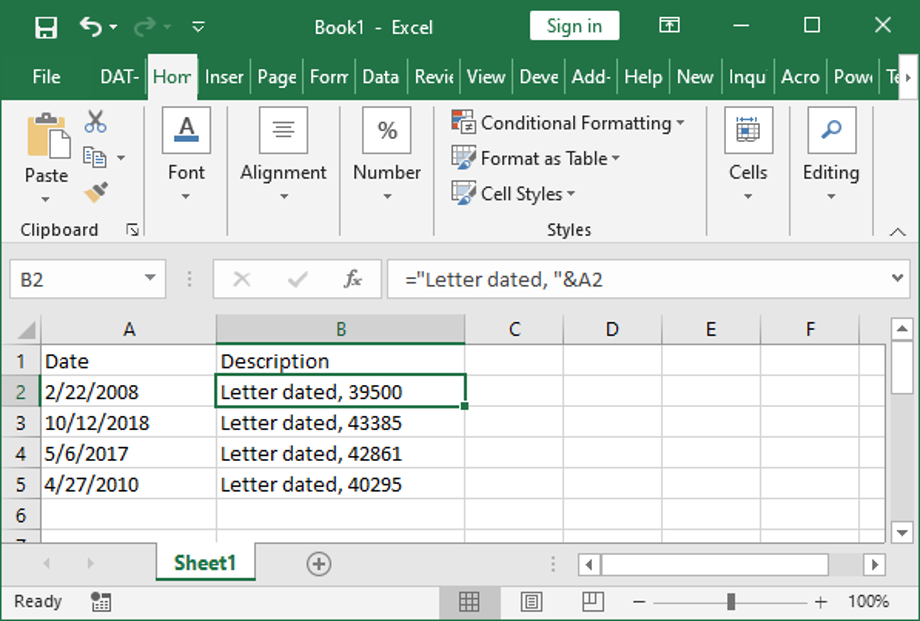top of pageSearch

# Excel TEXT Function to Display Date in Formula

Don't miss that in Excel when you want to reference a date in another column (that is formatted as a date, not as text) in a formula in another cell and display it in the correct date format, you can use the TEXT function.

In this example we see that when a reference is made to a date in a formula using a simple cell reference, we get the date converted to a number.If instead the formula is written using the TEXT function:

="Letter dated, "&TEXT(A2,"mm/dd/yyyy")

. . . the date will be shown in the result of formula.bottom of page